# A relation between the second moment of a distribution and one of its particular probability

I had recently posted a question here: To prove a relation involving a probability distribution

The relations quoted in the above question are used extensively in fluid mechanics and many other fields, but no one has provided any proof for that. The relations (as stated in my previous question) are:

$$\begin{equation} \alpha + p_6 = 1, \quad \quad \quad 0.66 < p_6 < 1, \end{equation}$$ $$\begin{equation} \alpha p_6^2 = 1 / 2 \pi, \quad \quad \quad 0.34 < p_6 < 0.66, \end{equation}$$

where $$p_n = \frac{e^{-c_1 n - c_2/n}}{Z},$$ and $$c_1$$ and $$c_2$$ are constants and Z is a normalization factor, also $$n \geq 3$$. $$\alpha$$ (the second moment) is defined as $$\alpha = \sum_{n = 3}^{\infty} p_n (n - 6)^2$$, where it is assumed that $$\langle n \rangle = 6$$.

The answer to my previous question shows that these relations are not correct exactly and the use of equality sign in some papers in the literature is not quite correct. My question is: Is it possible to obtain these formulas as some sort of approximate relations? The main problem is that how one could get rid of the constants in $$p_n$$ and obtain such a general law? (If it is possible at all!)

EDIT

As mentioned in the comments, the same relations are obtained here, see Eq.(16); however, their probability function, Eq.(8), is different from the one which is mentioned in the question. Since in the most papers, the authors have used the $$p_n$$ as mentioned in my question, one guesses that these relations are also derivable from the above $$p_n$$. The approach of the mentioned book is to construct a generating function and recast the problem into a Mayer series. Then by keeping the first few terms one can obtain the above relations; however, this approach, as far as I can see, is not applicable to the above $$p_n$$.

• "are used extensively in fluid mechanics" --- do you have a few sources? Jul 13, 2021 at 11:08
• @CarloBeenakker Thanks for your comment. I will add sources in a few minutes.
– user318428
Jul 13, 2021 at 11:56
• @CarloBeenakker For example in this one: journals.aps.org/pre/pdf/10.1103/PhysRevE.101.042309 Eq.(8) is the distribution which I have quoted. It says in general one can obtain a relation between the second moment and $p_6$ and have cited papers. In this paper, they have not quoted the above relations explicitly, but one can find in the others (I will also cite these papers).
– user318428
Jul 13, 2021 at 12:02
• tandfonline.com/doi/abs/10.1080/13642819308220137 in page 355, in the second paragraph, they have used the first relation.
– user318428
Jul 13, 2021 at 13:39
• @CarloBeenakker In Eq.(1) of the following, they have used the second relation in my question: mdpi.com/2073-4352/10/8/703/htm
– user318428
Jul 13, 2021 at 14:11

As in the previous answer, the equality $$\alpha + p_6 = 1$$ can be rewritten as $$\sum_{n=3}^\infty g(n)p_n=1,$$ where $$g(n):=(n-6)^2+1(n=6)\ge1(n\in\{5,6,7\})+4\times1(n\notin\{5,6,7\}).$$ Therefore and because $$p_n>0$$ for all $$n\ge3$$, we have $$\sum_{n=3}^\infty g(n)p_n>\sum_{n=3}^\infty p_n=1,$$ so that the equality $$\alpha + p_6 = 1$$ is always false.

It is also clear now that, for the equality $$\alpha + p_6 = 1$$ to hold approximately, we need the probabilities $$p_n$$ for $$n\notin\{5,6,7\}$$ to be negligible compared with $$p_n$$ for $$n\in\{5,6,7\}$$. Since $$p_n=e^{h(n)}/Z$$ with $$h(n):=-c_1n-c_2/n$$ and the mean $$\sum_{n\ge3}np_n$$ is $$6$$, we see that (for the equality $$\alpha + p_6 = 1$$ to hold approximately), the $$p_n$$'s hence should peak near $$6$$, that is, $$h$$ should peak near $$6$$. Since $$h'(6)=-c_1+c_2/6^2$$ and $$h''(6)=-2c_2/6^3$$, we see that $$c_2\approx 36 c_1$$ and $$c_2$$ must be quite large. Vice versa, for such $$c_1$$ and $$c_2$$, the peaking condition will hold and hence we will have $$\alpha + p_6\approx1$$. Such conditions on $$c_1$$ and $$c_2$$ ($$c_2\approx 36 c_1$$ with $$c_2$$ quite large) apparently hold in the in fluid mechanics settings of interest.

As an illustration, here is the discrete plot $$\{(n,p_n)\colon n\in\{3,\dots,10\}\}$$ for $$c_1=10$$ and $$c_2=36\times10$$:In this case, $$p_6=0.72368\dots$$ and $$\alpha + p_6=1.0149\dots$$.

Concerning the second (approximate) identity, $$\begin{equation} \alpha p_6^2 \approx 1 / 2 \pi, \tag{2} \end{equation}$$ note that, if $$h$$ sharply peaks at $$6$$, then $$h$$ is approximately quadratic near $$6$$, and hence (cf. Carlo Beenakker's answer) $$\begin{equation} p_n\approx e^{-(n-6)^2/(2s^2)}/S(s) \tag{!} \end{equation}$$ for some real $$s>0$$, where $$S(s):=\sum_{n=-\infty}^\infty e^{-(n-6)^2/(2s^2)}=\sum_{n=-\infty}^\infty e^{-n^2/(2s^2)} =\vartheta _3\left(0,e^{-1/(2 s^2)}\right)$$ and $$\vartheta$$ is the elliptic theta function. Next, $$\alpha\approx S_2(s)/S(s),$$ where $$S_2(s):=\sum_{n=-\infty}^\infty(n-6)^2e^{-(n-6)^2/(2s^2)} =\sum_{n=-\infty}^\infty n^2e^{-n^2/(2s^2)}=s^3 S'(s).$$

So, the approximate identity (2) can be rewritten as $$R(s):=2\pi S_2(s)/S(s)^3\approx1$$ and would of course follow from the exact identity $$\begin{equation} R(s)=1, \tag{*} \end{equation}$$ which seems to be true at least for $$s\ge1$$, in view of the graphs $$\{(s,R(s))\colon 1/10 (below) and $$\{(s,1-R(s))\colon 1/10 (further below):In turn, (*) would follow from the exact identity $$\begin{equation} S(s)=s\sqrt{2\pi}, \tag{**} \end{equation}$$ which seems to be true at least for $$s\ge1.5$$, in view of the graph $$\{(s,\frac{S(s)}{s\sqrt{2\pi}}-1)\colon 1/10 below:However, at this point I am not familiar enough with the theta function to prove identity (*) or (**).

Update: As was pointed out to me (and of which I should have thought myself) -- despite the dramatic graphs, neither one of the identities (*) and (**) can hold exactly for all $$s$$ in any (say) nonempty open interval $$I\subset(0,\infty)$$. Indeed, otherwise they would hold for all $$s\in(0,\infty)$$, because the function $$S$$ can be analytically continued to the angle domain $$\{z\in\mathbb C\colon\Re(z^2)>0\}$$. However, for $$s\downarrow0$$ we have $$S(s)\to1\ne s\sqrt{2\pi}$$ and $$S_2(s)\to0$$, whence $$R(s)\to0\ne1$$.

Yet, by the Poisson summation formula, for $$s\to\infty$$, $$\begin{equation} S(s)=s\sqrt{2\pi}\sum_{k=-\infty}^\infty e^{-2k^2 \pi^2 s^2}\sim s\sqrt{2\pi} \end{equation}$$ and $$\begin{equation} S_2(s)=s^3\,\sqrt{2\pi}\sum_{k=-\infty}^\infty e^{-2k^2 \pi^2 s^2}(1-4k^2 \pi^2 s^2)\sim s^3\,\sqrt{2\pi}, \end{equation}$$ whence $$R(s)\sim1$$ -- so that the approximate identity (2) holds for large enough $$s>0$$.

Next, large $$s>0$$ correspond to a (somewhat) low degree of peakedness of $$(p_n)$$, whereas the "discrete Gaussian" approximation (!) works only when $$(p_n)$$ is sufficiently peaked. Thus, the approximate identity (2) holds when $$(p_n)$$ is peaked (at $$n=6$$) but not too much -- that is, when $$p_6$$ is large enough but not too close to $$1$$. The particular numbers such as $$0.34$$ and $$0.66$$ in the OP's formula (2) play no special role except to affect the closeness of the approximation.

• @Loi : In the condition $0.66 < p_6 < 1$, the number $0.66$ plays no special role. The closer $p_6$ to $1$, the closer $\alpha+p_6$ to $1$. E.g.,, for $c_1=5$ and $c_2=36\times 5$, we have $p_6=0.51\dots$ and $\alpha+p_6=1.16\dots$. Jul 13, 2021 at 14:01
• I understood that as $p_6$ approaches $1$, $\alpha + p_6$ also approaches $1$. I was asking this question as based on the relations in my question, if $p_6$ is less than $0.66$ the second relation is a better approximation, as it is claimed in the literature.
– user318428
Jul 13, 2021 at 14:04
• Also, Carlo Beenakker in the comments has posted this link which the authors interestingly have obtained both relations mathematically, see Eq.(16): books.google.nl/… Their $p_n$, Eq.(8), is different from mine in the question, so I don't think their approach is directly applicable, but it shows both relations are mathematically derivable!
– user318428
Jul 13, 2021 at 23:37
• I have added a consideration of the second approximate identity. Jul 15, 2021 at 4:44
• I have added an update that seems to completely solve the mystery of the second approximate identity. Jul 15, 2021 at 11:50

Simple argument for Lemaître's law.

Let me first give a simple argument for the relation $$2\pi\alpha p_6^2=1$$ between the variance $$\alpha$$ and the probability $$p_6$$ (a relation known as Lemaître's law).

I approximate the distribution $$p_n$$ by a Gaussian $$P(n)$$ centered at $$n=6$$ and ignore the discreteness of $$n$$. I also ignore the restriction to $$n\geq 3$$ and allow $$n$$ to range from $$-\infty$$ to $$+\infty$$. This may be justified by the expectation that only values of $$n$$ close to the peak at 6 will contribute to the first few moments, provided that $$p_6$$ is not too small.

So I replace $$p_n$$ by $$P(n)=\frac{1}{\sqrt{2\pi\alpha}}e^{-(n-6)^2/2\alpha},$$ and the relation $$2\pi\alpha P(6)^2=1$$ follows.

The reason that this relation fails if $$p_6$$ becomes close to unity, is that then the discreteness of $$n$$ cannot be neglected -- since only three values $$n=5,6,7$$ contribute. For $$p_6$$ near 1 that then gives the relation $$\alpha+p_6=1$$ derived by Iosif Pinelis.

Numerical test of Lemaître's law.

The simple argument given above is crude and needs to be justified. For that purpose I have tested it numerically, for the distribution $$p_n = Z^{-1}e^{-c_1 n - c_2/n},\;\;n\geq 3,\;\;\text{with}\;\;Z=\sum_{n=3}^\infty e^{-c_1 n - c_2/n},$$ subject to the constraint that $$\bar{n}=6$$.

I show plots of $$\alpha = \sum_{n = 3}^{\infty} p_n (n - 6)^2$$ versus $$p_6$$ (blue data points) and compare with the two expressions $$\alpha=(2\pi p_6^2)^{-1}$$ (red) and $$\alpha=1-p_6$$ (green). The agreement is quite remarkable, without any fitting parameter. The lower range $$p_6>0.34$$ mentioned in the OP can be relaxed to smaller values of $$p_6$$, the agreement between blue and red remains good for $$p_6\gtrsim 0.2$$. The crossover from red to green indeed happens near $$p_6=0.66$$.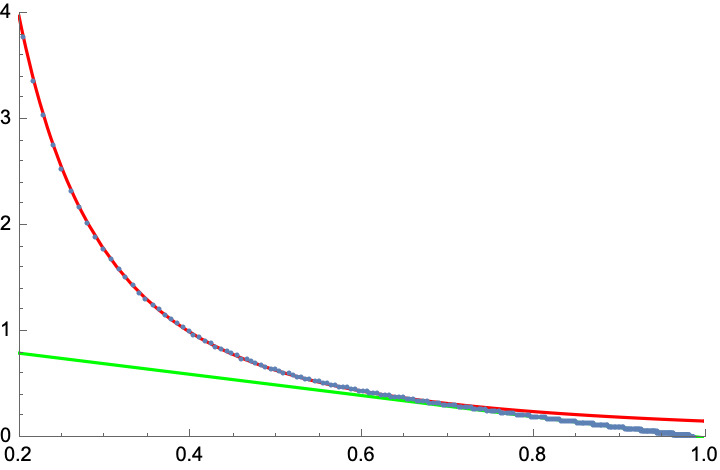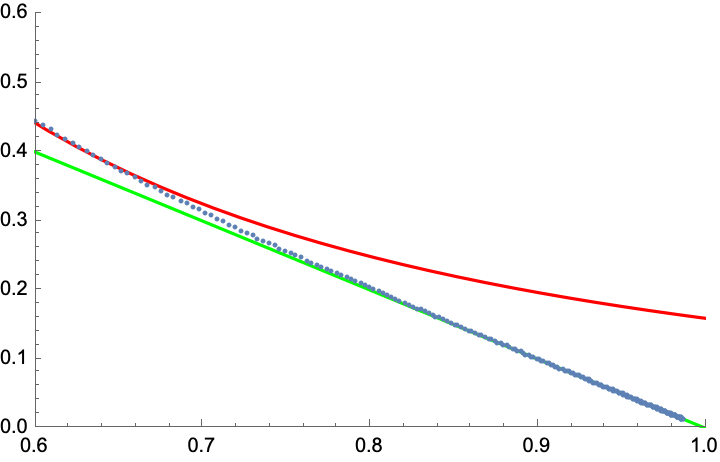Let me also compare with the $$p_n$$ used by Rivier, $$p_n = Z^{-1}e^{c_1 n - c_2 n^2},\;\;n\geq 3,\;\;\text{with}\;\;Z=\sum_{n=3}^\infty e^{c_1 n - c_2 n^2},$$ again subject to the constraint that $$\bar{n}=6$$.

Now the deviation between blue and red indeed starts at larger $$p_6$$, close to the value 0.34 in the OP.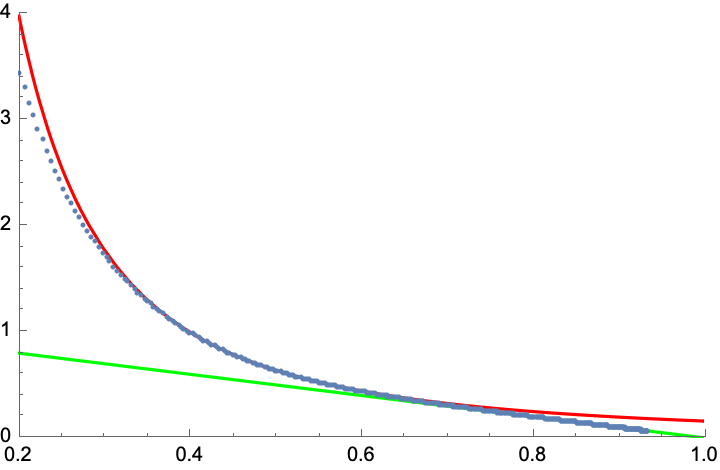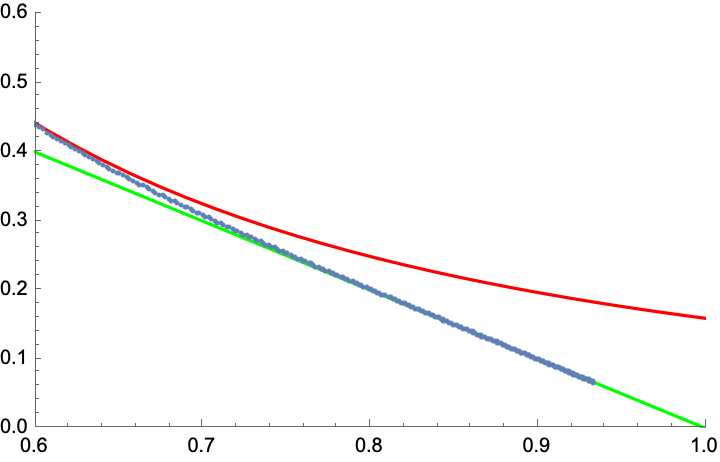Here is a test of the relation $$c_2=36 c_1$$ mentioned by Iosif Pinelis (data points in blue), for the first $$p_n$$ (left plot), and also a plot of $$p_6$$ versus $$c_1$$ (right plot):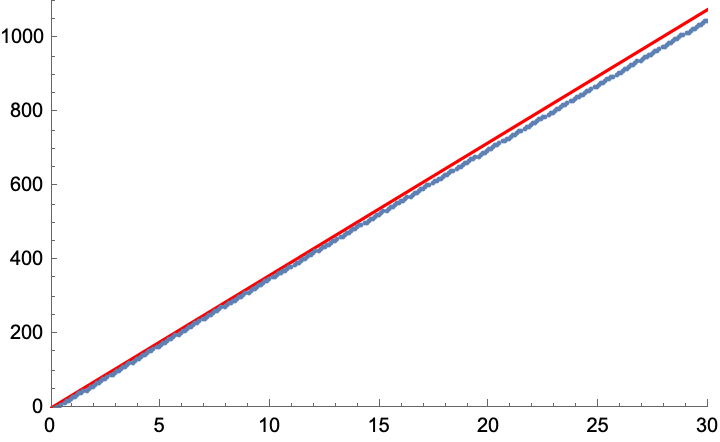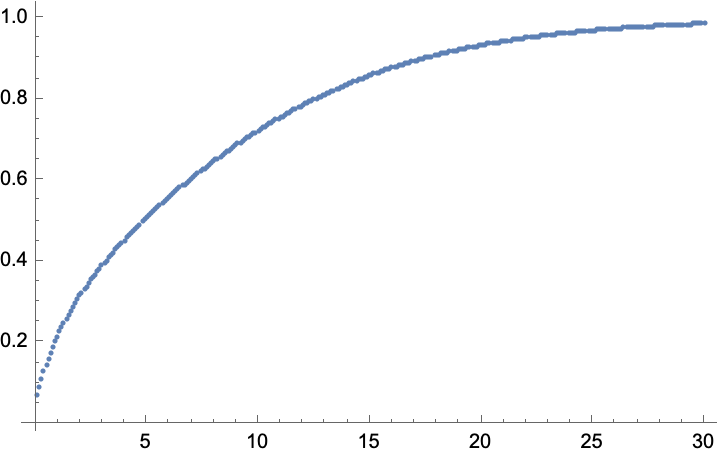For the second $$p_n$$, the analogous relation is $$c_2=c_1/12$$, and this is indeed very close to the data points: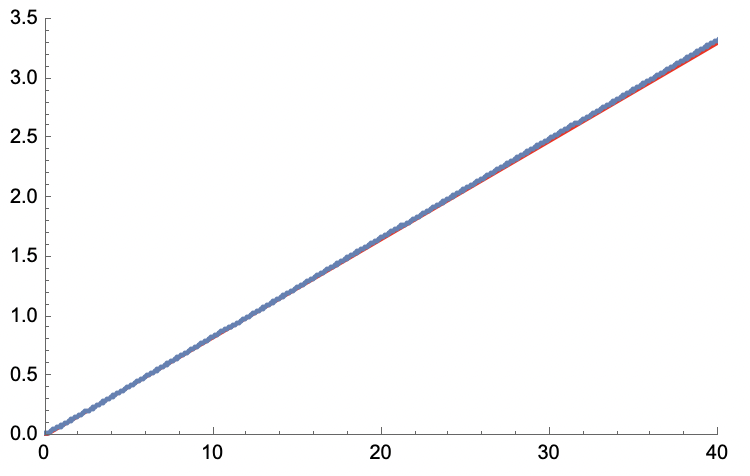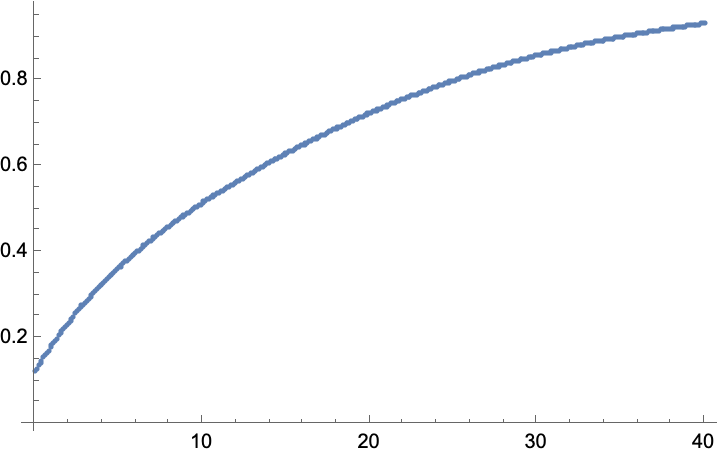The right plot again shows $$p_6$$ as a function of $$c_1$$.

• Thanks for this analysis! Besides the very strange generality of these relations, it's quite interesting that the crossover from red to green occurs near $0.66$ which implies these relations are also valid for the $p_n$ in my question and this is puzzling as one can obtain same relations for completely two different $p_n$'s, one in the mentioned book by you and one in my question.
– user318428
Jul 14, 2021 at 19:37
• Also, what values did you choose for $c_1$ and $c_2$? Are they in agreement with the other answer which says $c_2$ should be large and $c_2 = 36 c_1$?
– user318428
Jul 14, 2021 at 19:45
• In the Rivier $p_n$, is it $c_1$ or $-c_1$, just I want to check if it's not a typo.
– user318428
Jul 14, 2021 at 19:55
• I added the test for the relation $c_2=36c_1$, for the distribution in the posted question, it agrees quite well; as for the sign of $c_1$ in the second distribution: Rivier has written the distribution in an equivalent, but slightly different form, with factors $n-6$ and $(n-6)^2$; with the choice I wrote down the coefficient in front of the linear term is positive. Jul 14, 2021 at 20:00
• a cross-over, not a phase transition (this number "0.66" is not sharply defined) Jul 15, 2021 at 10:35